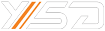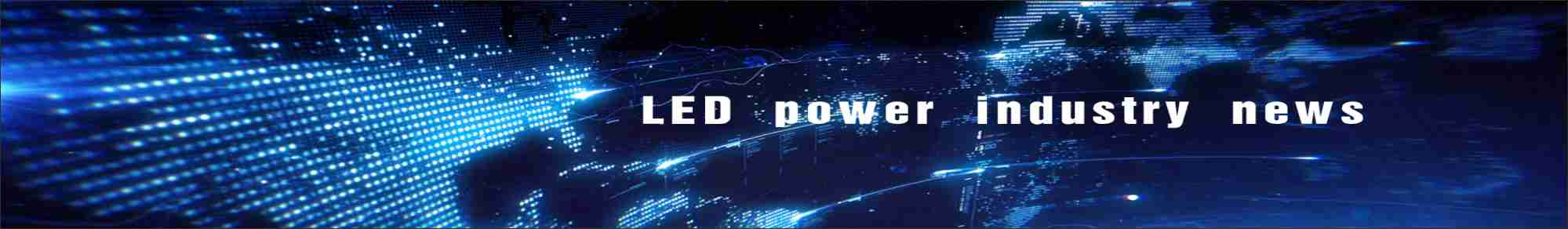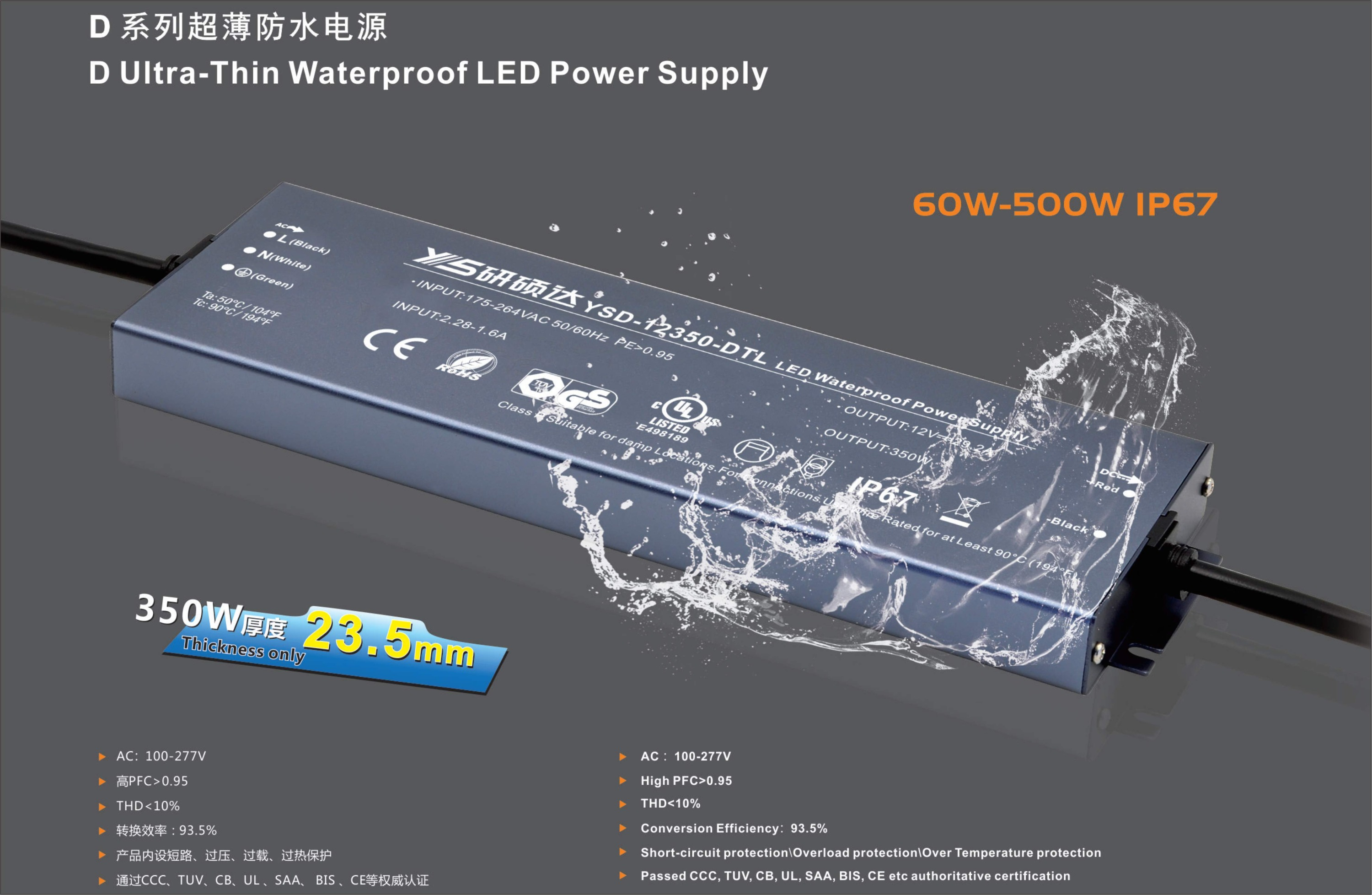#The importance of high power factor in LED driver power supply
Your Position：Home>News>Industry-news>The importance of high power factor in LED driver power supply

# What is the power factor?

Power factor refers to the ratio of the active power of the AC circuit to the apparent power.

Under a certain voltage and power, the higher the value, the better the efficiency, and the more fully the power generation equipment can be utilized.

The size of the Power Factor is related to the load nature of the circuit, such as incandescent bulb, resistance furnace and other resistance load of Power Factor is 1, generally has the Power Factor of inductive load circuit is less than 1.Power factor is an important technical data of power system.Power factor is a coefficient to measure the efficiency of electrical equipment.Low power factor indicates that the reactive power of the circuit used for alternating magnetic field conversion is large, thus reducing the utilization rate of the equipment and increasing the power supply loss of the line.In AC circuits, the cosine of the phase difference between voltage and current (Φ) is called the power factor, expressed by the symbol cos N, in numerical terms, the power factor is the ratio of active power and apparent power, that is, cos D =P/S.

# High power factor power generation background

The traditional AC-DC transformation obtains the DC voltage by means of capacitor filtering through the rectifying circuit of the AC grid. The main disadvantages of this transformation circuit are:

(1), the input AC voltage is a sine wave, but the input AC current is a pulse current, waveform serious distortion, interference with the grid voltage, to generate radiation around and along the wire transmission of electromagnetic interference;

(2), In order to get the adjustable DC voltage, the thyristor controllable rectifier circuit is adopted, but the ripple is very large, and a large filter is needed to get the stable DC voltage.

In addition, AC current contains a large number of harmonic current, so that the current waveform in the grid is seriously distorted, the input power factor of the power supply is low, and the utilization efficiency is reduced.

# Measures to improve the power factor

A filter inductor LZ is connected between the filter capacitors of the rectifier to increase the conductive width of the input AC current, slow down the current impact, reduce the waveform distortion, and thus reduce the harmonic component of the current.

(2),Active PFC

Active power factor correction, referred to as APFC, mainly controls the input current to be sine wave change, and the phase difference between the input voltage is as close as possible to 0, that is, the power factor is close to 1.# The importance and benefits of high power factor (PF)

The power factor is the ratio of the actual power consumed to the power supply capacity, so the higher the power factor is, the less unnecessary loss can be reduced and the utilization rate of power can be improved in the process of power retransmission.The energy problem in the field of relighting in developed countries has been attached great importance. For example, European energy standard EVP5 and American energy star have clearly stipulated in this respect that the power factor PF of residential lighting driver must be greater than 0.7 and that of commercial lighting must be greater than 0.9.YSD High-end LED Driver Power, PFC>0.95%, THD<10%, built-in EMI filter circuit, 100% full load high temperature aging.Products are exported to Europe, Australia, America and so on.